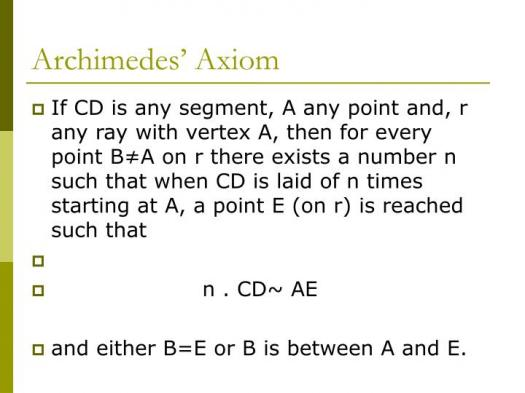# What Do You Know About Axiom Of Archimedes?

10 Questions | Total Attempts: 111SettingsThanks to the Axiom V of Archimedes’ On the Sphere and Cylinder, the axiom of Archimedes (or Archimedean axiom, Archimedes' axiom, Archimedes' lemma, or the continuity axiom) is one of the most widespread concepts in mathematical analysis and the field of abstract algebra. It states that one magnitude can find a multiple of either of two magnitudes which will exceed the other, given the magnitudes have a ratio.

• 1.
Who gave the axiom of Archimedes its name?
• A.

Otto Stolz

• B.

Reynald Harvey

• C.

Paul Autz

• D.

Charles Puma

• 2.
Which structure holds the principle?
• A.

Linear structure

• B.

Algebraic structure

• C.

Trigonometric structure

• D.

Exponential structure

• 3.
What is the property of the element?
• A.

Infinitely small

• B.

Large

• C.

Equal

• D.

Small

• 4.
What do we call a structure in which a pair of non-zero elements is infinitesimal with respect to the other?
• A.

Non-Archimedean

• B.

Archimedean

• C.

Linear-Archimedean

• D.

Algebraic-Archimedean

• 5.
What do we call a structure in which neither of the non-zero elements is infinitesimal with respect to the other?
• A.

Non-Archimedean

• B.

Archimedean

• C.

Linear-Archimedean

• D.

Algebraic-Archimedean

• 6.
The theory plays an important role in which of the following?
• A.

Global field

• B.

Exponential functions

• C.

Local field

• D.

Trigonometric functions

• 7.
Which property must be Archimedean in the context of ordered field?
• A.

Field of the trigonometric functions

• B.

Field of real numbers

• C.

Field of integers

• D.

Field of rational functions

• 8.
Which property must be non-Archimedean in the context of ordered field?
• A.

Field of the trigonometric functions

• B.

Field of real numbers

• C.

Field of integers

• D.

Field of rational functions

• 9.
In which decade was the concept named? ​​​​The...
• A.

1870s

• B.

1880s

• C.

1890s

• D.

1900s

• 10.
For linearly ordered group G, what do x and y denote?
• A.

Positive elements

• B.

Negative elements

• C.

Integers

• D.

Real numbers

Related TopicsBack to top